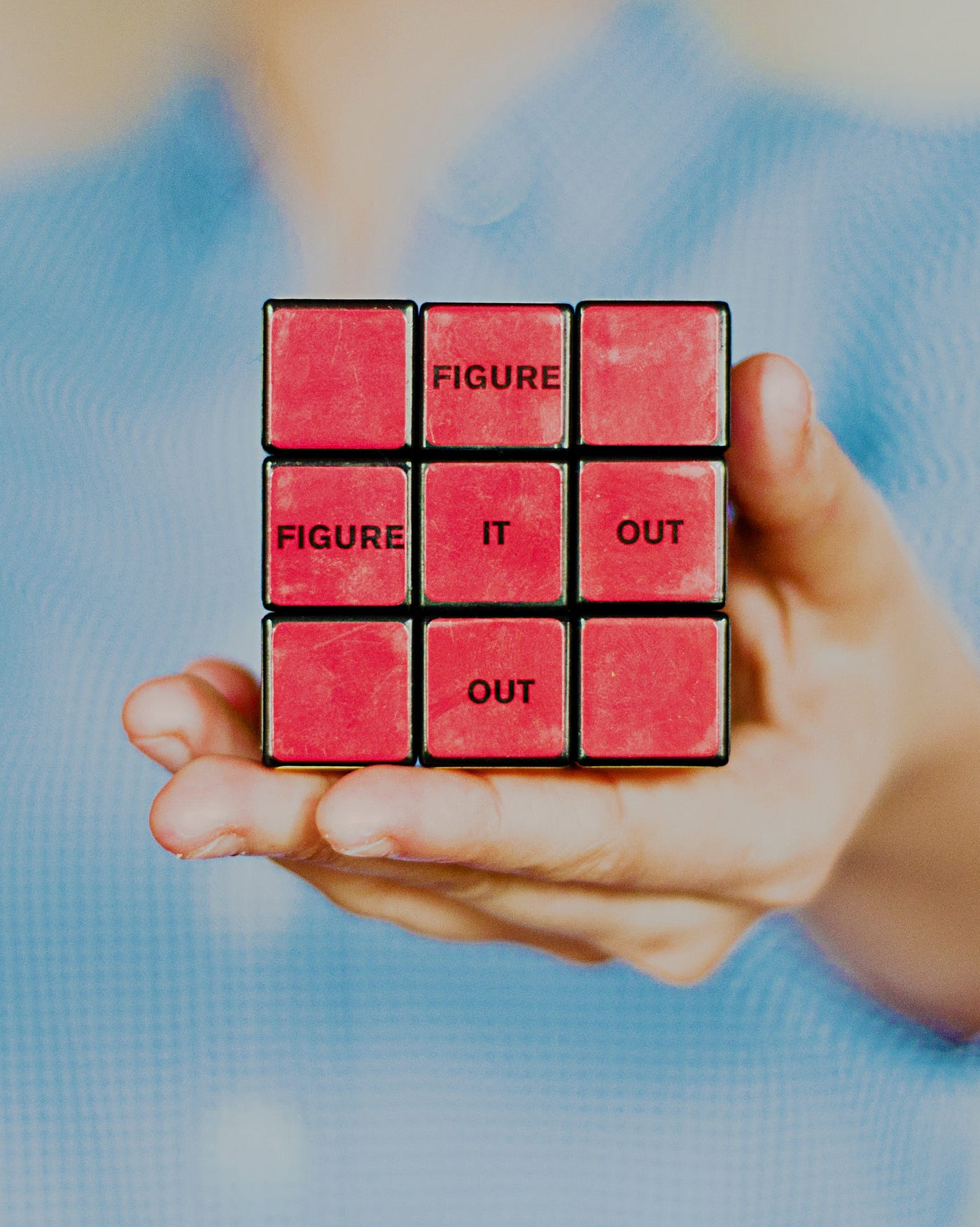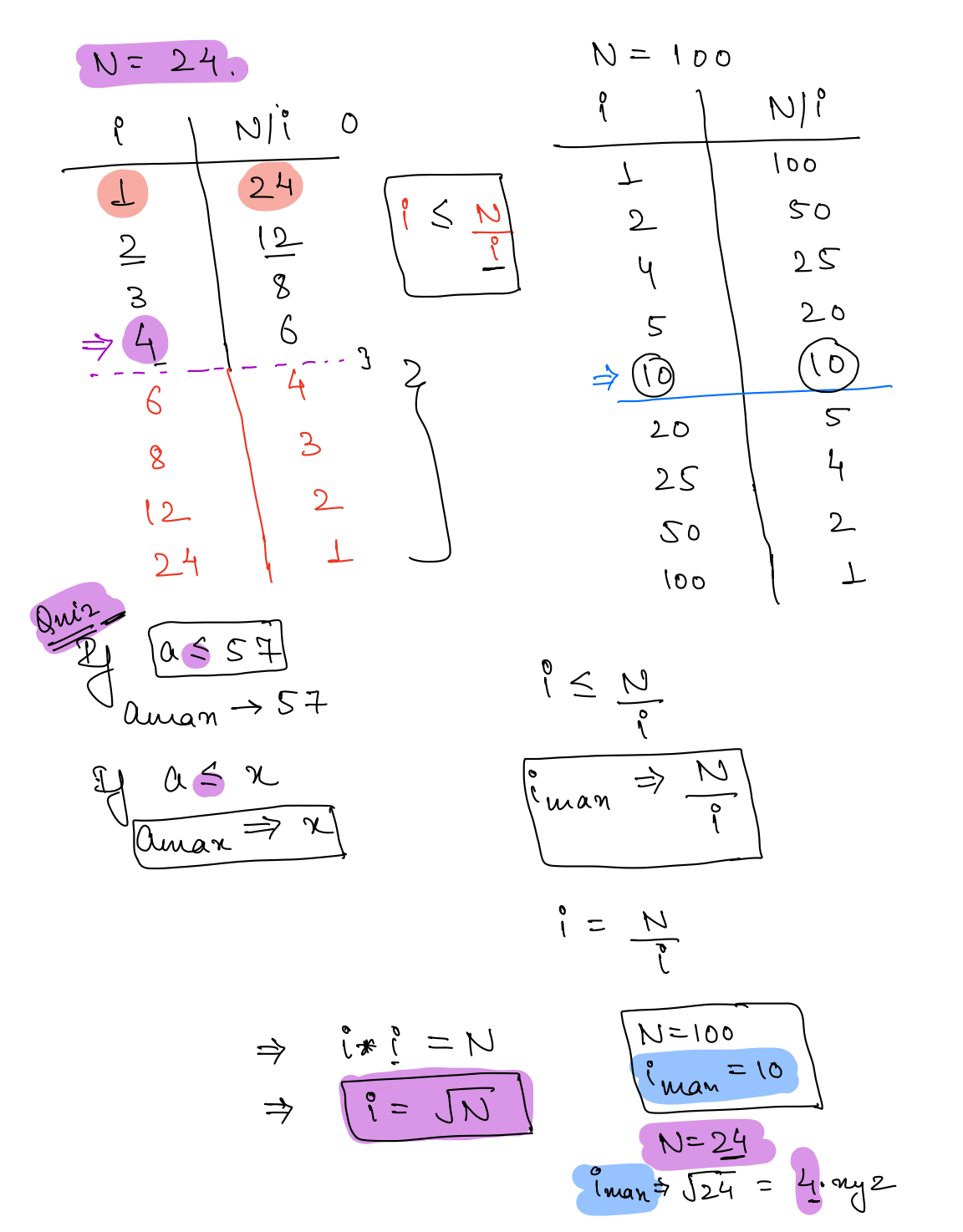# Introduction to Problem SolvingPhoto by Karla Hernandez on Unsplash

In this chapter we will see why we need a better approach to solve a problem.

Question : Given a number check whether it is Prime Or not.
e.g 15 , 12, 9, 23
23 is a prime
Prime Number: Any positive number that has exactly two factors, 1 and number(N) itself.

Pseudo code for the same:

Here we can see number of iterations are N i.e we are ranging i from [1-N]

Assumption:
10⁸ iterations takes 1 sec to process in our computer.
i.e 1 iterations will take 1/10⁸ sec.
if N has a value in terms of 10⁹ it will take 10 sec (10⁹/10⁸=10)
if N has a value in terms10¹⁸ it will take 10¹⁰ sec which is approximately 317 years →not feasible.As we can see factors are always appearing in pair, so instead of checking from 1-N we can check till 1-root(N)

pseudo code for the Approach 2:(based on the above observation)

Now again going back to our assumption :
10⁸ iterations takes 1 sec to process in our computer.
if N=10¹⁸ in approach 2 we are iterating i from 1 to till root(10¹⁸) i.e 10⁹.
So 10⁹ iterations will take 10 sec, and we have come down from 317 years to 10 sec.

As you can see that’s pretty much a optimisation that we did for this problem.

--

--